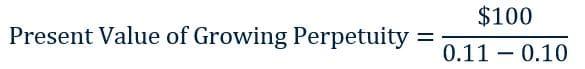#### This video shows how to calculate the present value of a growing perpetuity using a formula. A perpetuity refers to a series of cash flows that will continue forever. If the amount of the cash flow increases each period, we refer to it as a growing perpetuity. Because a dollar received in the future is worth less than a dollar received today (due to the time value of money), we discount a growing perpetuity to its present value. The video provides an example to show how this is done.

A perpetuity is a series of cash flows that will continue forever.  If the amount of the cash flow increases each period, we refer to it as a growing perpetuity.  Because a dollar received in the future is worth less than a dollar received today (due to the time value of money), we discount the cash flows from the growing perpetuity to their present value.  The following formula will allow you to calculate the present value of a growing perpetuity:where:

r = the discount rate

g = the growth rate (the rate at which the cash flows will increase each period)

Initial Cash Flow = the first cash flow in the series of perpetuities

Let’s say we’d like to take the present value of a growing perpetuity where the initial cash flow is \$100, the discount rate is 11%, and the growth rate is 10% (the growth rate means the cash flow in the second year will be \$110, in the third year will be \$121, etc.).  The present value of this stream of cash flows would be:We converted the percentages to decimals to perform the calculations.  After solving the problem above, we obtain a present value of \$10,000.  Thus, if your discount rate is 11% and someone offers you an infinite series of cash flows that start at \$100 and increase by 10% each period, the value of this stream of cash flows to you in present dollars is \$10,000.